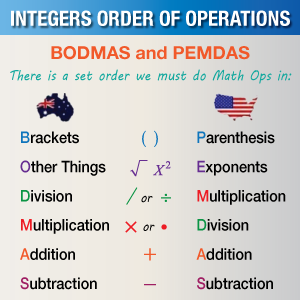# BODMAS

Go back to  'Multiplication and Division'

## Introduction to BODMAS

Grammar, just like mathematics plays a vital role in our daily lives. Sentences with punctuations at different parts of the statement could change the entire meaning upon delivery. Depending on what word is enunciated, the whole sentence can be played out differently. For example, a sentence with just a comma in the middle could imply two different things.

## “Don’t drink, and drive safely.”

Punctuations define and give structure to a sentence, guiding the reader on what words or phrases to enunciate. Similarly, the rules of BODMAS outline the order of the operations and give structure to nested operations.

## The importance of BODMAS

Without proper structure, every problem statement could produce two or more different answers, based on how the problem solver views the equation. Taking a simple problem statement into example;

A person spent Rs. 500 on food during 5 days of the week. On one of those days, he ordered an addition Rs. 200 worth of food. What was his total expenditure on food?

Mathematically, the statement comes out to be:

5 x 500 + 200

There could be at the most two possible outcomes, the answers could either be 2700 or 3500. The answers depend on the mathematical operation you choose to begin with first. In this case, there is no right answer.

So, how do we get to the right answer? The solution lies in the structuring of the statement representing the problem. To bring universal order and structure to the way problems or equations with nested statements are solved, BODMAS is used.

### Breaking down BODMAS

BODMAS is an acronym for;

• Bracket (parenthesis) o You always begin with anything that is inside Brackets.

• 5 x (500 + 200)

5 x 700 = 3500

• Orders (powers and square roots, cube roots) o Following B, the next in line are Orders. You perform them before moving on to the next mathematical operation.

• 32 + 6 = ?

You begin with performing the power sum first and then adding 6 to the result.

3 x 3 = 9 + 6 = 15

• Division and Multiplication (left to right) o Going from left to right, after you have finished with the B and O part of the equation, you move on to Division and Multiplication. Division and Multiplication rank equally, so whichever operation comes first from left to right, you calculate it first and then move on.

• 5 x  6 ÷ 3 + 8 = ?

You begin with the multiplying 5 x 6 = 30. Then you divide 30 by 3 and adding the result to 8.

Making it 30 ÷ 3 = 10 + 8 = 18

• Addition Subtraction (left to right). The last step is the Addition and Subtraction. Just like D and M, they rank equally. You perform whatever operation comes first from left to right.

• 5 + 7 – 8 + 3 = ?

You begin with adding 5 + 7 = 12. Next would be subtracting 8 from 12 and adding the result to 3.

Making it 12 – 8 = 4 + 3 = 7

An example with all the rules of BODMAS

(112 + 276) – 50 ÷ 52 + 18

2. Your sum now reads 388 – 50 ÷ 52 + 18

3. Next, orders or powers. In this case, that’s 52 = 25

4. The sum now reads 388 – 50 ÷ 25 + 18

5. Next, division and multiplication. There is no multiplication, but the division is 50 ÷ 25 = 2

7. Although you still have two operations left, addition and subtraction rank equally, so you just go from left to right. 388 - 2 = 386, and 386 + 18 = 404

(112 + 276) - 50 ÷ 52 + 18 = 404.

Another name for BODMAS is PEMDAS. They are essentially similar, just a difference in names due to where each term originated from. The following image will clear things up:### Errors during performing BODMAS

The presence of multiple brackets usually causes a state of confusion among the students.

They don't know which bracket to tackle first, leading to the generation of an incorrect answer.

We use this equation as an example of multiple brackets

4 + 3[8 –2(6 – 3)] ÷ 2

We begin with working from the inside of the brackets. Tackling the innermost bracket first and then moving outside. Starting with

6 – 3 = 3 4 + 3[8 – 2(3)] ÷ 2

Next, we work with the order. Thereby multiplying

2 (3) or 2 x 6 = 6 4 + 3[8 – 6] ÷ 2

There is one bracket left, [8 – 6] = 2

4 + 3 ÷ 2

There is still one more order to work with 3  or 3 x 2 = 6

4 + 6 ÷ 2

Post B and O comes D, hence, 6 ÷ 2 = 3

4 + 3

And lastly, addition. 4 + 3 = 7

4 + 3[8 –2(6 – 3)] ÷ 2 = 7

Going by the rule of BODMAS guarantees that you will not make an error while calculating to obtain the final result. But in case of confusion, make sure to write each step neatly and one below the other to prevent messing up any order. Keep going back to each step to make sure that you have not missed any operations.